# Horizontal barplot with R and ggplot2

A nice looking horizontal barplot with several customizations to explore the number of infections caught in laboratories made with `R` and `ggplot2`. This blogpost guides you through a step-by-step construction of a horizontal barplot that includes a variety of custom color fonts, labels, and annotations

Thanks to them for all the inspiring and insightful visualizations! Thanks also to Tomás Capretto who replicated the chart in R! 🙏🙏

As a teaser, here is the plot we’re gonna try building:At first sight, one may be tempted to think that today’s chart looks rather simple. However, it actually contains several subtle customizations that when added all together make the final result look beautiful and original. This is also going to be a great opportunity to use the `grid` library, the drawing library behind `ggplot2`, and `shadowtext`, a library that allows us to draw text with shadows.

``````library(grid)
library(tidyverse)

# Create data

Let’s get started by creating the objects that are going to hold the data for us. Note these values are inferred from the original plot and not something we computed from the original data source.

``````names <- c(
"Hantavirus", "Tularemia", "Dengue", "Ebola", "E. coli",
"Tuberculosis", "Salmonella", "Vaccinia", "Brucella"
)

# Name is an ordered factor. We do this to ensure the bars are sorted.
data <- data.frame(
count = c(6, 7, 7, 9, 11, 15, 17, 18, 54),
name = factor(names, levels = names),
y = seq(length(names)) * 0.9
)``````

And let’s also define the colors:

``````# The colors
BLUE <- "#076fa2"
RED <- "#E3120B"
BLACK <- "#202020"
GREY <- "grey50"``````

# Basic barchart

Creating a horizontal basic barchart with ggplot2 is quite simple. You use `geom_col()` passing the `count` variable to the first `aes()` variable, and `name` to the second one. Then, you can also use a different `fill` and `width`, as below:

``````plt <- ggplot(data) +
geom_col(aes(count, name), fill = BLUE, width = 0.6)

plt``````# Customize layout

The next step is to customize the layout: modify the axes configuration, change the color of the background, remove the tick marks, add grid lines, change the fonts, and more. Sounds like many things to change? Come on, it’s not going to bee too hard!

``````plt <- plt +
scale_x_continuous(
limits = c(0, 55.5),
breaks = seq(0, 55, by = 5),
expand = c(0, 0), # The horizontal axis does not extend to either side
position = "top"  # Labels are located on the top
) +
# The vertical axis only extends upwards
scale_y_discrete(expand = expansion(add = c(0, 0.5))) +
theme(
# Set background color to white
panel.background = element_rect(fill = "white"),
# Set the color and the width of the grid lines for the horizontal axis
panel.grid.major.x = element_line(color = "#A8BAC4", size = 0.3),
# Remove tick marks by setting their length to 0
axis.ticks.length = unit(0, "mm"),
# Remove the title for both axes
axis.title = element_blank(),
# Only left line of the vertical axis is painted in black
axis.line.y.left = element_line(color = "black"),
# Remove labels from the vertical axis
axis.text.y = element_blank(),
# But customize labels for the horizontal axis
axis.text.x = element_text(family = "Econ Sans Cnd", size = 16)
)

plt``````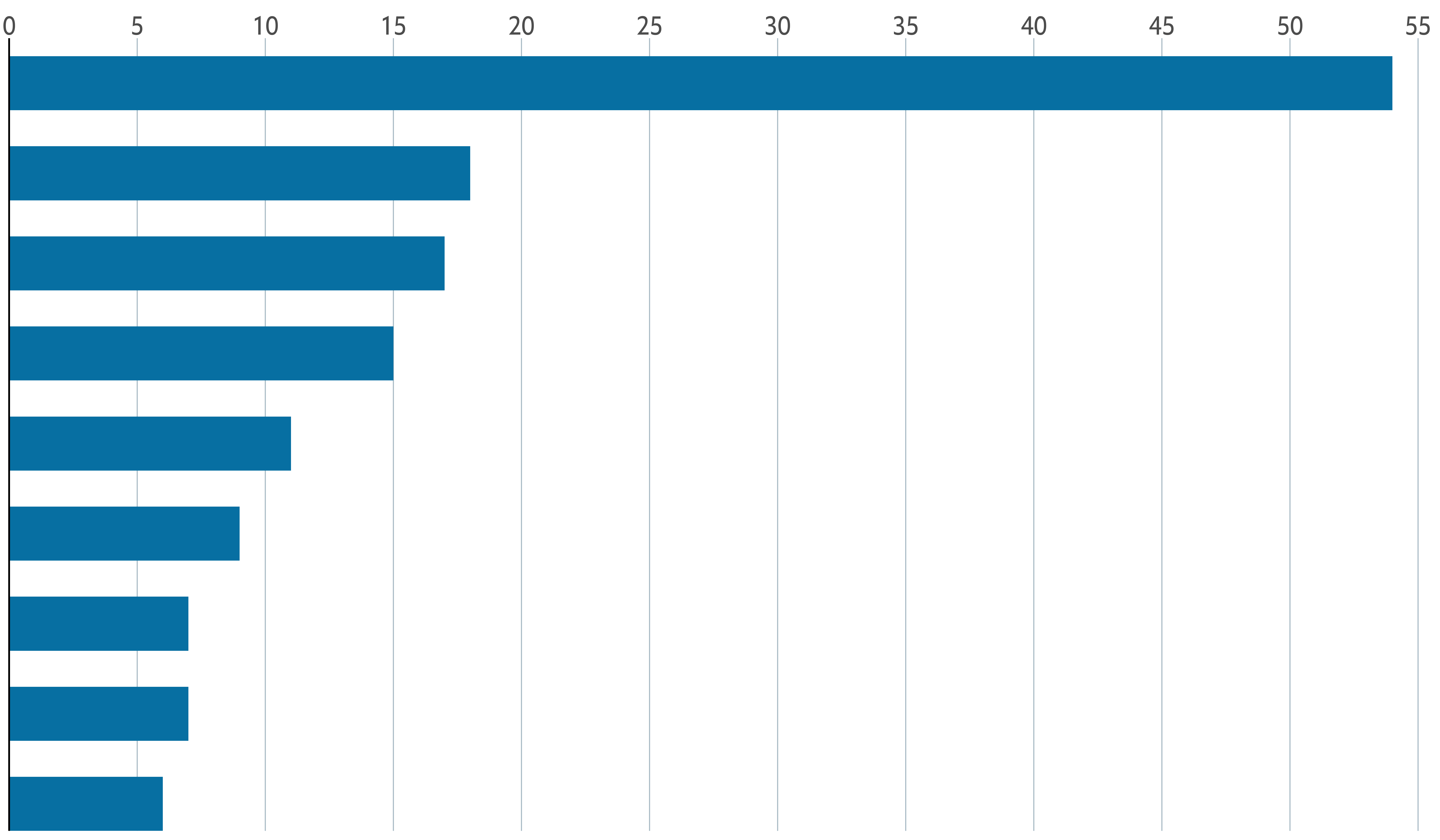Customizing the layout was a big step towards to the final chart. But there’s still work to be done.

So far, the chart doesn’t indicate anything about which disease is represented by each bar. Isn’t it frustrating? So now it is a good idea to add labels to the bars. Let’s do it!

The following chunk uses both `geom_text()` and `geom_shadowtext()`. The first one is used to draw regular text within the bars of the diseases with a count equal or above 8. On the other hand `geom_shadowtext()` is used to draw text with shadow to the right of the bars of the diseases with a count below 8. This shadow is a subtle but important detail that hides the grid line at 10 that passes behind the text.

``````plt <- plt +
data = subset(data, count < 8),
aes(count, y = name, label = name),
hjust = 0,
nudge_x = 0.3,
colour = BLUE,
bg.colour = "white",
bg.r = 0.2,
family = "Econ Sans Cnd",
size = 7
) +
geom_text(
data = subset(data, count >= 8),
aes(0, y = name, label = name),
hjust = 0,
nudge_x = 0.3,
colour = "white",
family = "Econ Sans Cnd",
size = 7
)

plt``````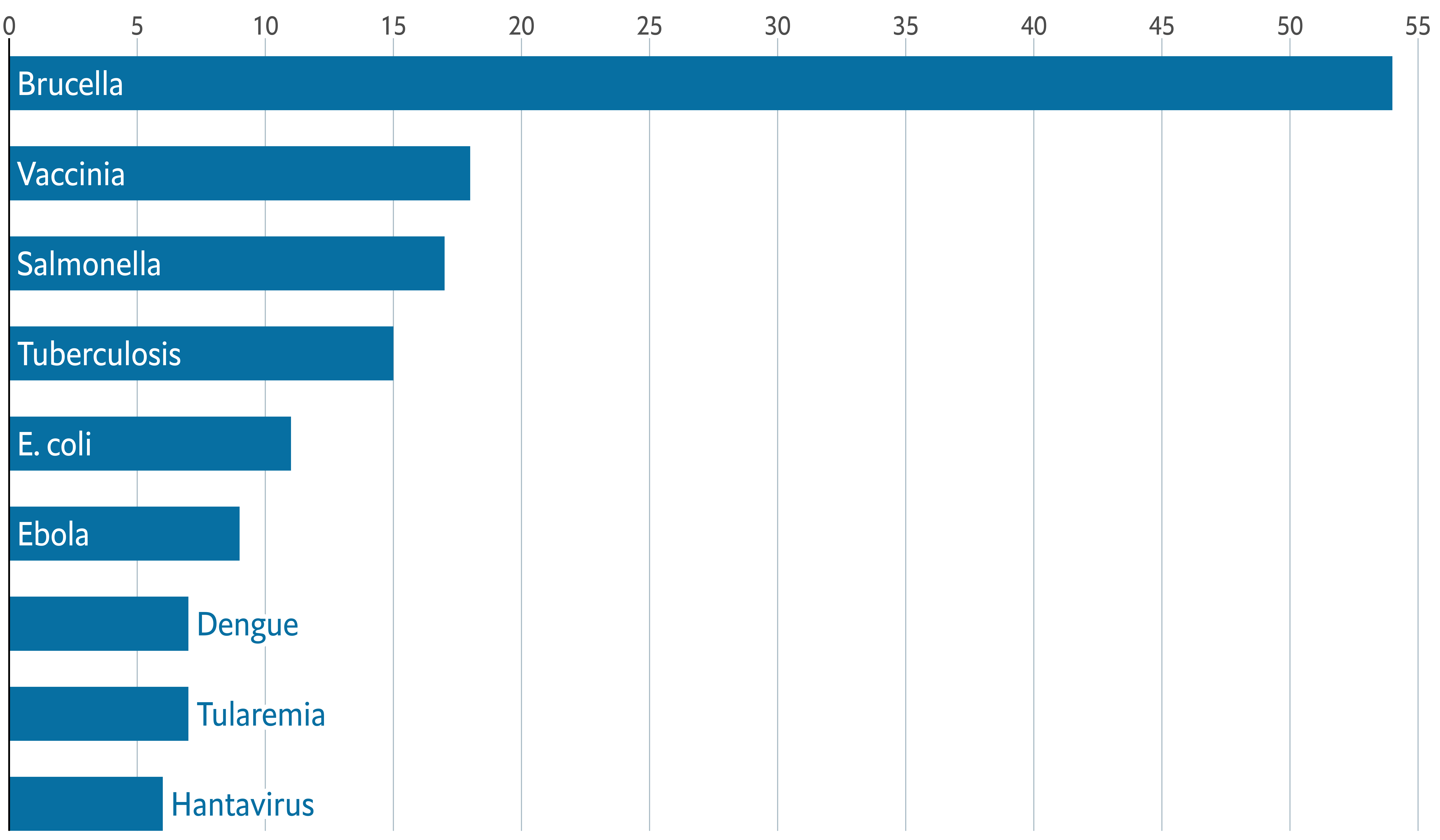# Add annotations and final tweaks

Adding titles is one of those steps that are simple from the technical point of view but can make a huge difference in the quality of the chart.

The next chunk shows how to add a title and a subtitle to a ggplot2 chart using the `labs()` function. Later, their aspect is customized using `theme()`.

``````plt <- plt +
labs(
title = "Escape artists",
subtitle = "Number of laboratory-acquired infections, 1970-2021"
) +
theme(
plot.title = element_text(
family = "Econ Sans Cnd",
face = "bold",
size = 22
),
plot.subtitle = element_text(
family = "Econ Sans Cnd",
size = 20
)
)
plt``````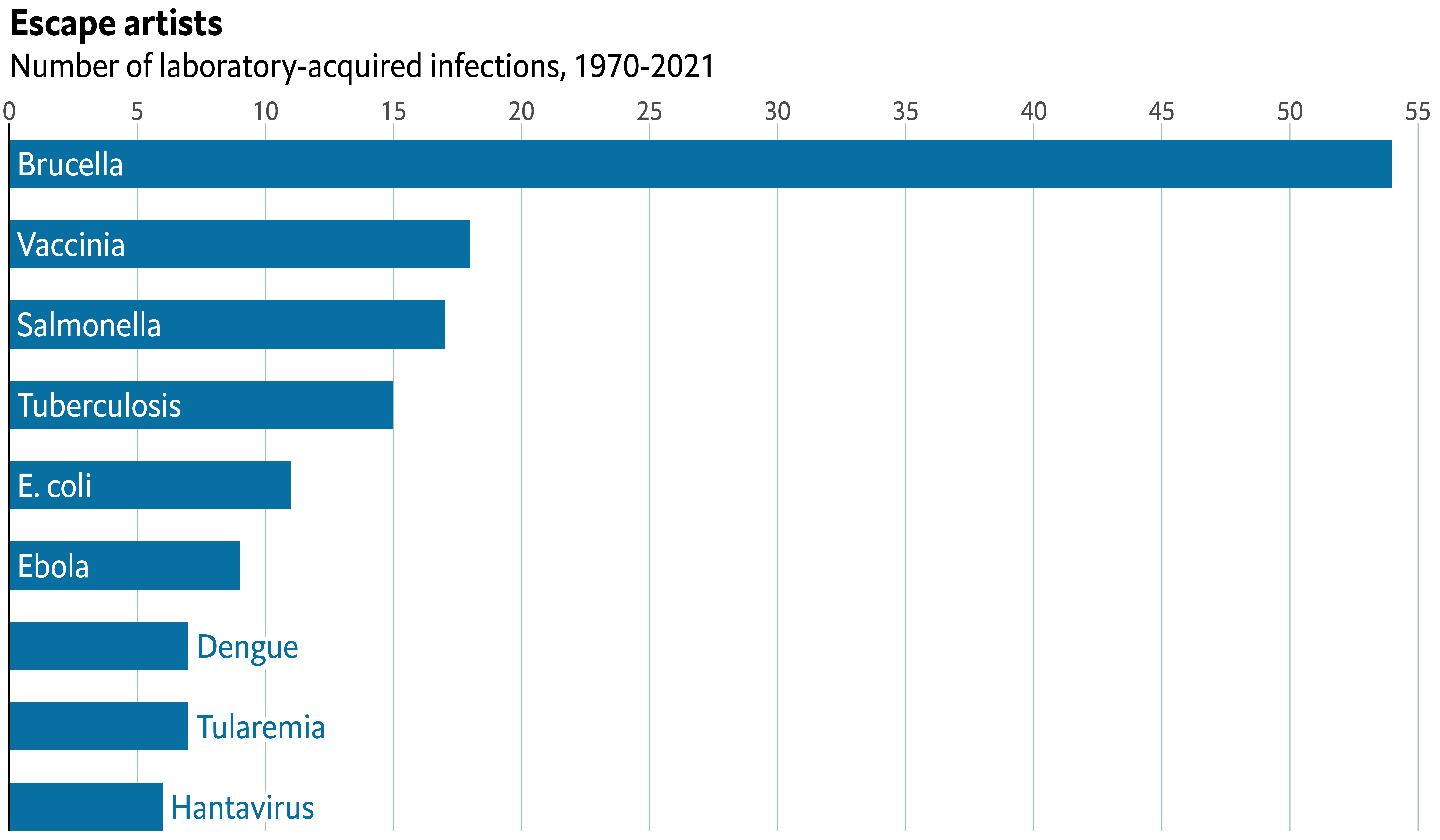This is how far we were able to get with ggplot2 alone. It’s quite evident the chart does not look like the original chart on top. It’s still missing the red line and rectangle on top, which is like a watermark of visualizations made by The Economist.

We’re going to use the `grid` library for this task. `grid` is a low-level plotting library that comes with any `R` installation by default and provides many plotting primitive functions. It is also the library that `ggplot2` uses to create the charts under the hood, and that’s why we can combine them in the same chart.

``````# Make room for annotations
plt <- plt +
theme(
plot.margin = margin(0.05, 0, 0.1, 0.01, "npc")
)

# Print the ggplot2 plot
plt

# Add horizontal line on top
# It goes from x = 0 (left) to x = 1 (right) on the very top of the chart (y = 1)
# You can think of 'gp' and 'gpar' as 'graphical parameters'.
# There we indicate the line color and width
grid.lines(
x = c(0, 1),
y = 1,
gp = gpar(col = "#e5001c", lwd = 4)
)

# lwd = 0 means the rectangle does not have an outer line
# 'just' gives the horizontal and vertical justification
grid.rect(
x = 0,
y = 1,
width = 0.05,
height = 0.025,
just = c("left", "top"),
gp = gpar(fill = "#e5001c", lwd = 0)
)

# We have two captions, so we use grid.text instead of
# the caption provided by  ggplot2.
grid.text(
"Sources: Laboratory-Acquired Infection Database; American Biological Safety Association",
x = 0.005,
y = 0.06,
just = c("left", "bottom"),
gp = gpar(
col = GREY,
fontsize = 16,
fontfamily = "Econ Sans Cnd"
)
)
grid.text(
"The Economist",
x = 0.005,
y = 0.005,
just = c("left", "bottom"),
gp = gpar(
col = GREY,
fontsize = 16,
fontfamily = "Milo TE W01"
)
)``````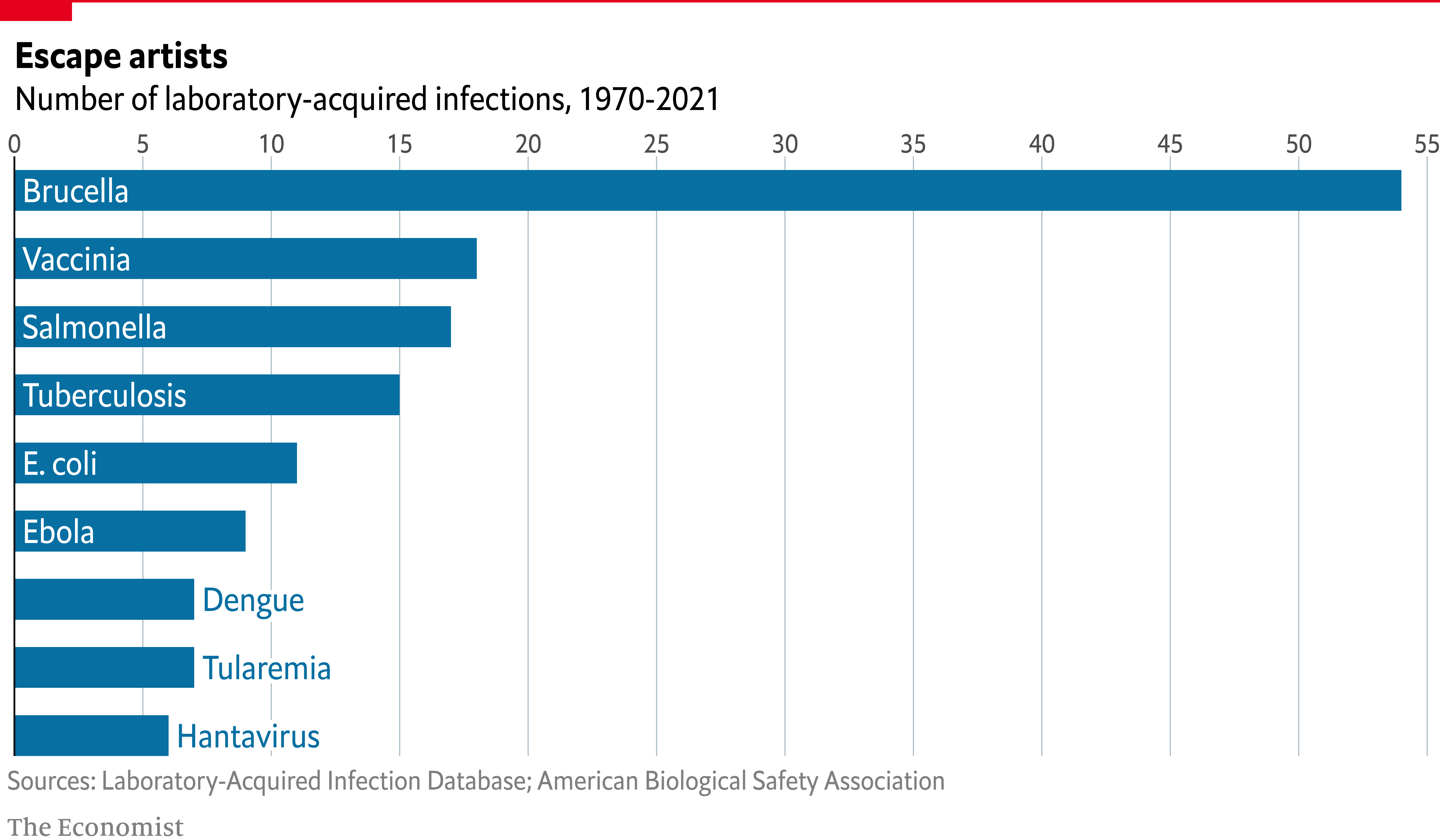Just comparing the sizes of the chunks you can see it definitely takes a considerable amount of extra work to get the details done. But in the end… Aren’t they’re worth it?

# The extra mile

If you are attentive to the smallest of the details you may have noticed the titles in the chart above aren’t aligned exactly in the same way than the titles in the original chart.

By default, `ggplot2` aligns titles using the panel region as reference. We asked ggplot2 to use the plot region as reference when we added `plot.title.position = "plot"` in the `theme()` call. But it was not enought. The title is not completely aligned to the left border of the chart, which you can notice by comparing the position of the rectangle on top with the titles.

This extra step consists of removing the titles generated with `ggplot2` and replacing them with text drawn with the `grix.text()` function.

``````plt <- plt +
labs(title = NULL, subtitle = NULL) +
theme(
plot.margin = margin(0.15, 0, 0.1, 0.01, "npc")
)

plt

grid.text(
"Escape artists",
0,
0.925,
just = c("left", "bottom"),
gp = gpar(
fontsize = 22,
fontface = "bold",
fontfamily = "Econ Sans Cnd"
)
)

grid.text(
"Number of laboratory-acquired infections, 1970-2021",
0,
0.875,
just = c("left", "bottom"),
gp = gpar(
fontsize = 20,
fontfamily = "Econ Sans Cnd"
)
)

grid.lines(
x = c(0, 1),
y = 1,
gp = gpar(col = "#e5001c", lwd = 4)
)

grid.rect(
x = 0,
y = 1,
width = 0.05,
height = 0.025,
just = c("left", "top"),
gp = gpar(fill = "#e5001c", lwd = 0)
)

grid.text(
"Sources: Laboratory-Acquired Infection Database; American Biological Safety Association",
x = 0.005,
y = 0.06,
just = c("left", "bottom"),
gp = gpar(
col = GREY,
fontsize = 16,
fontfamily = "Econ Sans Cnd"
)
)

grid.text(
"The Economist",
x = 0.005,
y = 0.005,
just = c("left", "bottom"),
gp = gpar(
col = GREY,
fontsize = 16,
fontfamily = "Milo TE W01"
)
)``````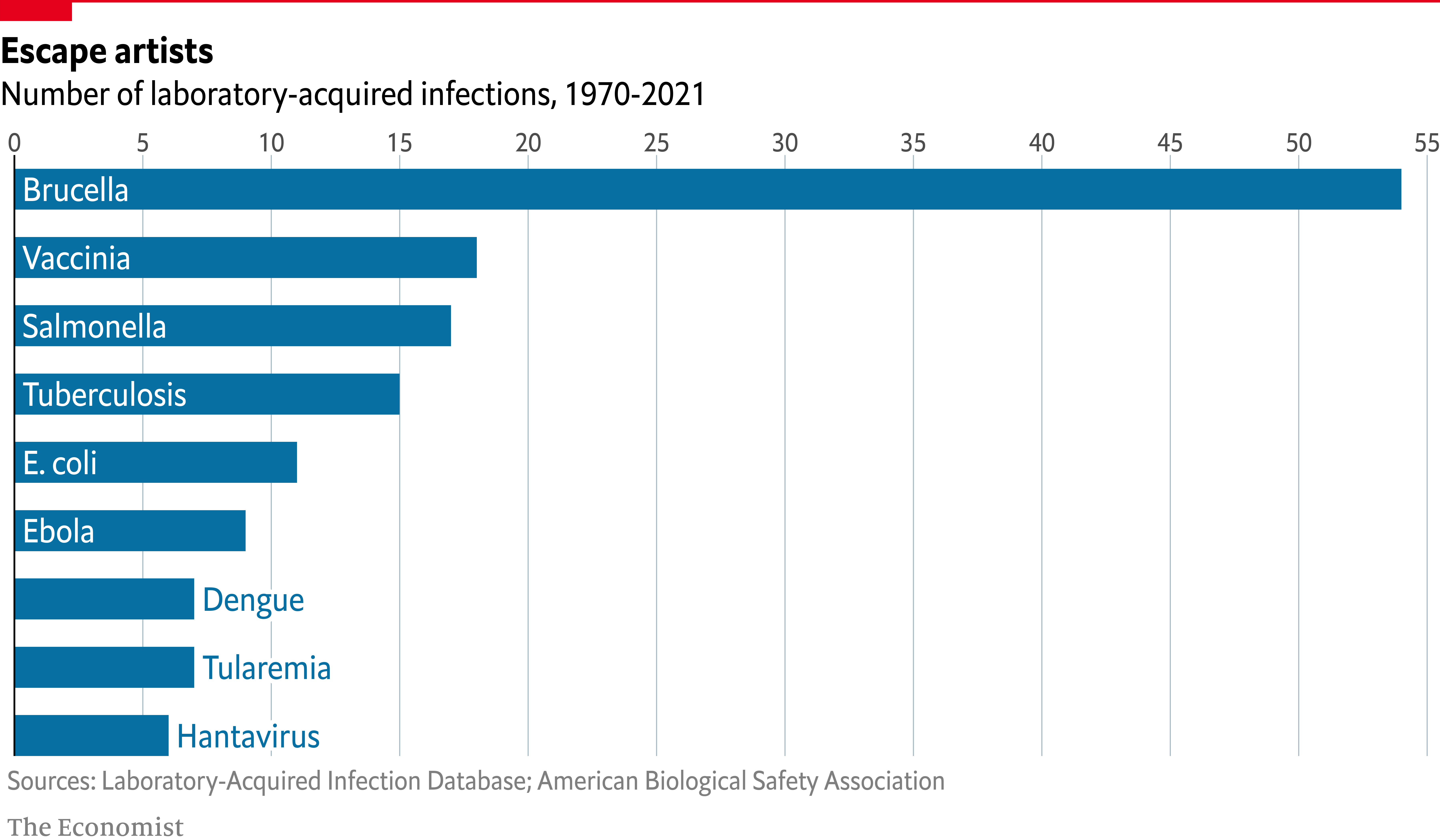It’s so satisfying to have nail it after so much work!

# Final notes

If you attempt to save the plot above with `ggsave()` you’ll end up with a file that only reflects what is done with `ggplot2`. The right way to save this chart that mixes `ggplot2` and `grid` is to use more low-level functions such as `png()`. For example

``````# Open file to store the plot
png("plot.png", width = 12, height = 7, units = "in", res = 300)

# Print the plot
plt

grid.text(
"Escape artists",
0,
0.925,
just = c("left", "bottom"),
gp = gpar(
fontsize = 22,
fontface = "bold",
fontfamily = "Econ Sans Cnd"
)
)

grid.text(
"Number of laboratory-acquired infections, 1970-2021",
0,
0.875,
just = c("left", "bottom"),
gp = gpar(
fontsize = 20,
fontfamily = "Econ Sans Cnd"
)
)

grid.lines(
x = c(0, 1),
y = 1,
gp = gpar(col = "#e5001c", lwd = 4)
)

grid.rect(
x = 0,
y = 1,
width = 0.05,
height = 0.025,
just = c("left", "top"),
gp = gpar(fill = "#e5001c", lwd = 0)
)

grid.text(
"Sources: Laboratory-Acquired Infection Database; American Biological Safety Association",
x = 0.005,
y = 0.06,
just = c("left", "bottom"),
gp = gpar(
col = GREY,
fontsize = 16,
fontfamily = "Econ Sans Cnd"
)
)

grid.text(
"The Economist",
x = 0.005,
y = 0.005,
just = c("left", "bottom"),
gp = gpar(
col = GREY,
fontsize = 16,
fontfamily = "Milo TE W01"
)
)

# Close connection
dev.off()``````

Related chart types

## Contact

This document is a work by Yan Holtz. Any feedback is highly encouraged. You can fill an issue on Github, drop me a message on Twitter, or send an email pasting yan.holtz.data with gmail.com.